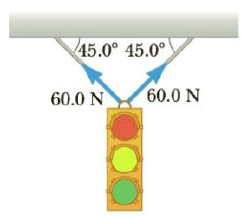Chapter 4, Problem 75AP

Chapter
Section
Textbook Problem

(a) What is the resultant force exerted by the two cables supporting the traffic light in Figure P4.75? (b) What is the weight of the light?Figure P4.75

(a)

To determine
The resultant force exerted by the cables.

Explanation

The horizontal components get cancelled. The vertical components get added up.

The free body diagram is given below.

From the diagram, the resultant force is,

FR=(120N)sin45ο=

(b)

To determine
The weight of the light.

Still sussing out bartleby?

Check out a sample textbook solution.

See a sample solution

The Solution to Your Study Problems

Bartleby provides explanations to thousands of textbook problems written by our experts, many with advanced degrees!

Get Started